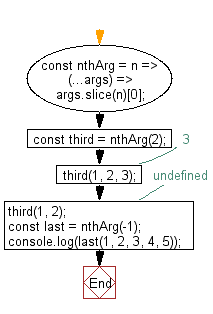# JavaScript: Create a function that gets the argument at index n. If n is negative, the nth argument from the end is returned

## JavaScript fundamental (ES6 Syntax): Exercise-92 with Solution

Write a JavaScript program to create a function that gets the argument at index n. If n is negative, the nth argument from the end is returned.

• Use Array.prototype.slice() to get the desired argument at index n.
• If n is negative, the nth argument from the end is returned.

Sample Solution:

JavaScript Code:

``````//#Source https://bit.ly/2neWfJ2
const nthArg = n => (...args) => args.slice(n);
const third = nthArg(2);
third(1, 2, 3); // 3
third(1, 2); // undefined
const last = nthArg(-1);
console.log(last(1, 2, 3, 4, 5));
```
```

Sample Output:

```5
```

Flowchart:Live Demo:

See the Pen javascript-basic-exercise-92-1 by w3resource (@w3resource) on CodePen.

Improve this sample solution and post your code through Disqus

What is the difficulty level of this exercise?

Test your Programming skills with w3resource's quiz.

﻿

## JavaScript: Tips of the Day

How to remove a specific item from an array?

Find the index of the array element you want to remove using indexOf, and then remove that index with splice.

The splice() method changes the contents of an array by removing existing elements and/or adding new elements.

```const array = [2, 5, 9];

console.log(array);

const index = array.indexOf(5);
if (index > -1) {
array.splice(index, 1);
}

// array = [2, 9]
console.log(array);```

The second parameter of splice is the number of elements to remove. Note that splice modifies the array in place and returns a new array containing the elements that have been removed.

For the reason of completeness, here are functions. The first function removes only a single occurrence (i.e. removing the first match of 5 from [2,5,9,1,5,8,5]), while the second function removes all occurrences:

```function removeItemOnce(arr, value) {
var index = arr.indexOf(value);
if (index > -1) {
arr.splice(index, 1);
}
return arr;
}

function removeItemAll(arr, value) {
var i = 0;
while (i < arr.length) {
if (arr[i] === value) {
arr.splice(i, 1);
} else {
++i;
}
}
return arr;
}
//Usage
console.log(removeItemOnce([2,5,9,1,5,8,5], 5))
console.log(removeItemAll([2,5,9,1,5,8,5], 5))
```

Ref: https://bit.ly/2N9nKRp